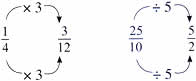Tuesday , July 5 2022# NCERT 5th Class (CBSE) Mathematics: Ratio And Proportion

## UNDERSTANDING RATIO

This necklace has been made by Annisha using red and yellow beads.

The ration of red beads to yellow beads is 5 : 4 0r 5/4

5 ( Number of red beads) /4 ( number of yellow beads )

The ration of yellow beads to red beads is 4 : 5 or 4/5

4 ( Number of yellow beads) /5 ( Number of red beads )

The ratio of yellow beads to all the beads is 4 : 9 or 4/9

4 ( Number of yellow beads) / 9 ( Total number of beads in the necklace )

The ration of red beads to all the beads is 5 : 9 or 5/9

5 ( Number of yellow beads) / 9 ( Total number of beads in the necklace )

### Equal Rations

Sunalini is making lime juice. She uses limes and sugar in the ration of 3 : 5 or 3/5

F0r one jug of juice she uses 3 limes and 5 spoons of sugar.

In case she wants to make 3 jugs of lime juice this is what she will do.

One jugs have lime to sugar in the ratio of 3 : 5 or 3/5

Two jugs have lime to sugar in the ratio of 6 : 10 or 6/10

Three jugs have lime to sugar in the ratio of 9 : 15 or 9/15

For three jugs of lime juice she needs 9 limes and 15 spoons of sugar.

She can also use the rules of fractions to find out how many limes and spoons of sugar she needs for three jugs.

For one jug  3 ( limes)/5 ( spoons of sugar ) or 3/5

For two jugs it will be 3 × 2/5 × 2 = 6/10

Similarly, for three jugs it will be 3 × 3/5 × 3 = 9/15

3/5 = 6/10 = 9/15

We can find equal rations by multiplying or dividing the terms by the same number like we do in fractions.We can use this relationship to find missing terms in equal ratios.

(a) 3 : 7 = ? : 14

3/7 = ?/14 Since 7 × 2 = 14, we should multiply 3 × 2 to get the answer.

3 × 2 / 7 × 2 = 6/14 and 3 : 7 = 6 : 14

(b) 32 : 24 = 4 : ?

32/24 = 4/? Since 32 ÷ 8 = 4, we should also divide 24 by 8 to get the answer.

32 ÷ 8 / 24 ÷ 8 = 4/3 and 32 : 24 = 4 : 3

## चुनौती हिमालय की 5th NCERT CBSE Hindi Rimjhim Ch 18

चुनौती हिमालय की 5th Class NCERT CBSE Hindi Book Rimjhim Chapter 18 प्रश्न: लद्दाख जम्मू-कश्मीर राज्य में …